# Completing the Square ALGEBRA 1 LESSON 9 5

• Slides: 10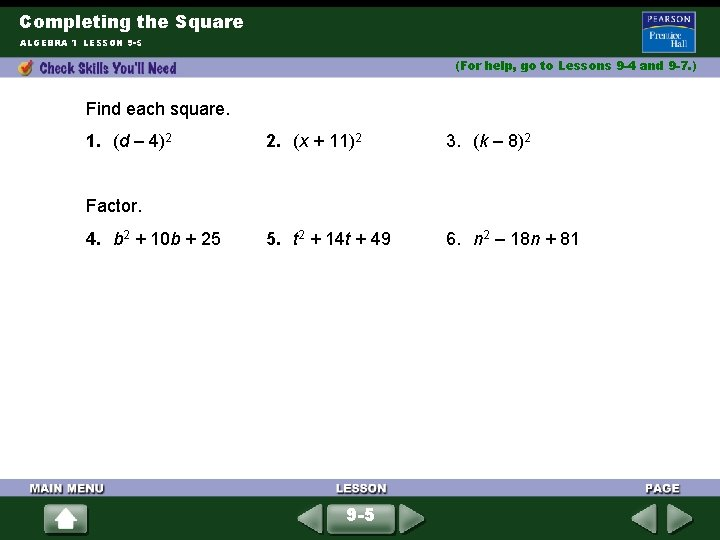Completing the Square ALGEBRA 1 LESSON 9 -5 (For help, go to Lessons 9 -4 and 9 -7. ) Find each square. 1. (d – 4)2 2. (x + 11)2 3. (k – 8)2 5. t 2 + 14 t + 49 6. n 2 – 18 n + 81 Factor. 4. b 2 + 10 b + 25 9 -5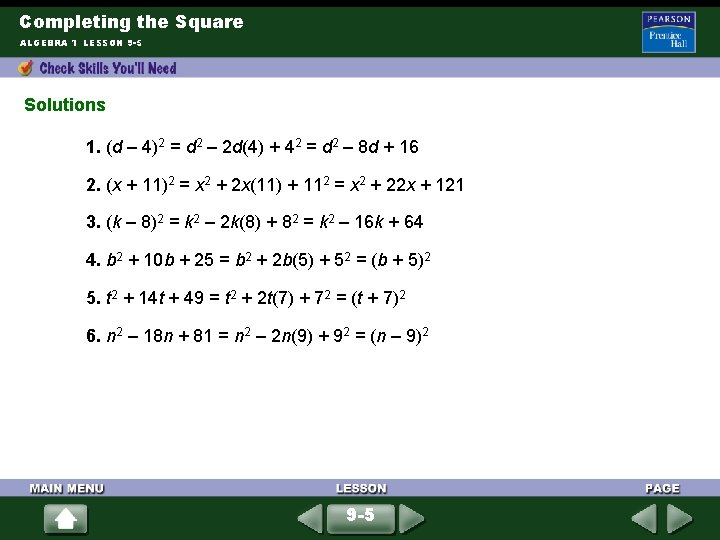Completing the Square ALGEBRA 1 LESSON 9 -5 Solutions 1. (d – 4)2 = d 2 – 2 d(4) + 42 = d 2 – 8 d + 16 2. (x + 11)2 = x 2 + 2 x(11) + 112 = x 2 + 22 x + 121 3. (k – 8)2 = k 2 – 2 k(8) + 82 = k 2 – 16 k + 64 4. b 2 + 10 b + 25 = b 2 + 2 b(5) + 52 = (b + 5)2 5. t 2 + 14 t + 49 = t 2 + 2 t(7) + 72 = (t + 7)2 6. n 2 – 18 n + 81 = n 2 – 2 n(9) + 92 = (n – 9)2 9 -5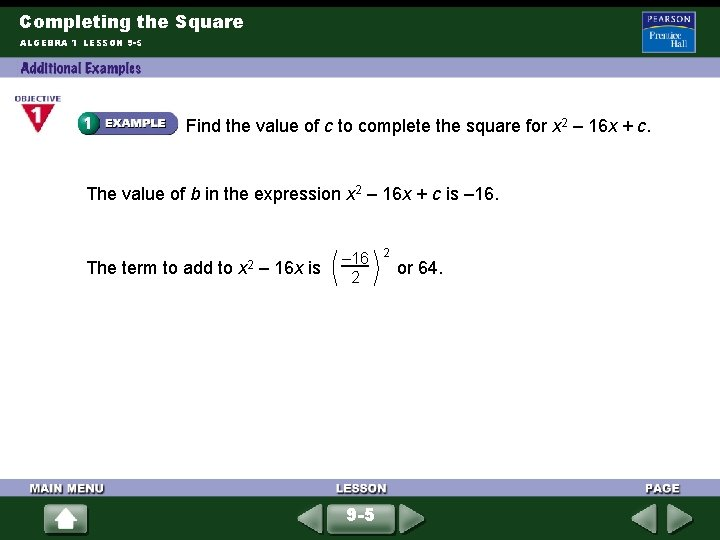Completing the Square ALGEBRA 1 LESSON 9 -5 Find the value of c to complete the square for x 2 – 16 x + c. The value of b in the expression x 2 – 16 x + c is – 16. The term to add to x 2 – 16 x is – 16 2 9 -5 2 or 64.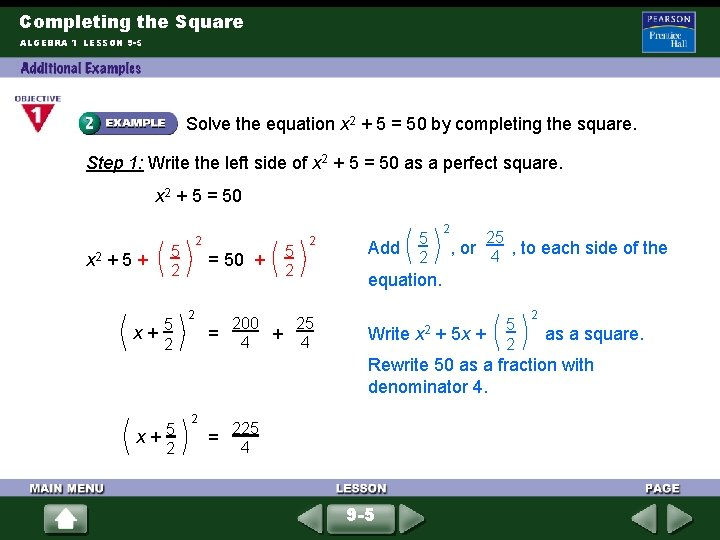Completing the Square ALGEBRA 1 LESSON 9 -5 Solve the equation x 2 + 5 = 50 by completing the square. Step 1: Write the left side of x 2 + 5 = 50 as a perfect square. x 2 + 5 = 50 x 2 + 5 + 2 5 2 x+5 2 = 50 + 2 5 2 2 200 25 = 4 + 4 Add 5 2 2 25 , or 4 , to each side of the equation. Write x 2 + 5 x + 5 2 2 as a square. Rewrite 50 as a fraction with denominator 4. x+5 2 2 = 225 4 9 -5Completing the Square ALGEBRA 1 LESSON 9 -5 (continued) Step 2: Solve the equation. 2 x+5 2 225 4 = ± Find the square root of each side. x + 5 = ± 15 2 x + 5 = 15 2 2 x=5 Simplify. 2 5 15 or x+2 = – 2 or x = – 10 Write as two equations. Solve for x. 9 -5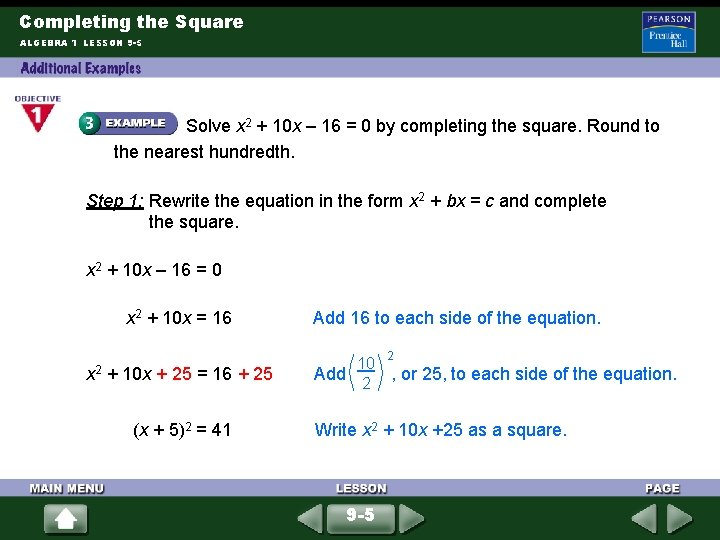Completing the Square ALGEBRA 1 LESSON 9 -5 Solve x 2 + 10 x – 16 = 0 by completing the square. Round to the nearest hundredth. Step 1: Rewrite the equation in the form x 2 + bx = c and complete the square. x 2 + 10 x – 16 = 0 x 2 + 10 x = 16 Add 16 to each side of the equation. 2 x 2 + 10 x + 25 = 16 + 25 (x + 5)2 = 41 10 Add 2 , or 25, to each side of the equation. Write x 2 + 10 x +25 as a square. 9 -5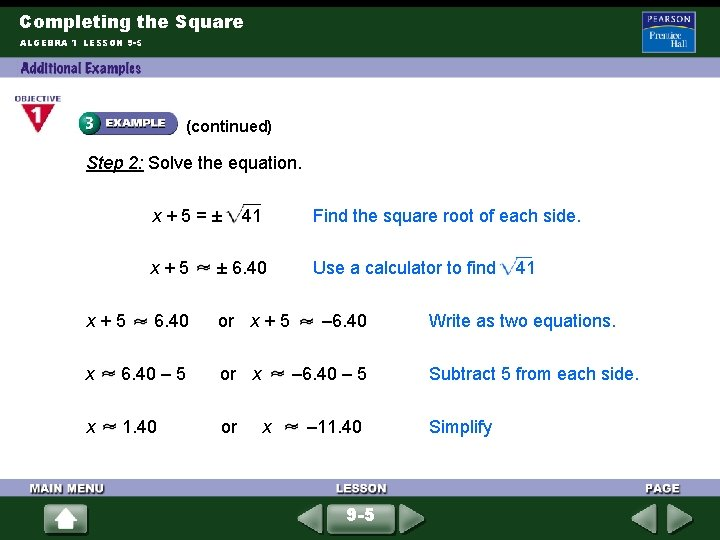Completing the Square ALGEBRA 1 LESSON 9 -5 (continued) Step 2: Solve the equation. x+5=± x+5 41 Find the square root of each side. x+5 ± 6. 40 or x + 5 x 6. 40 – 5 or x x 1. 40 or Use a calculator to find – 6. 40 – 5 x – 11. 40 9 -5 41 Write as two equations. Subtract 5 from each side. Simplify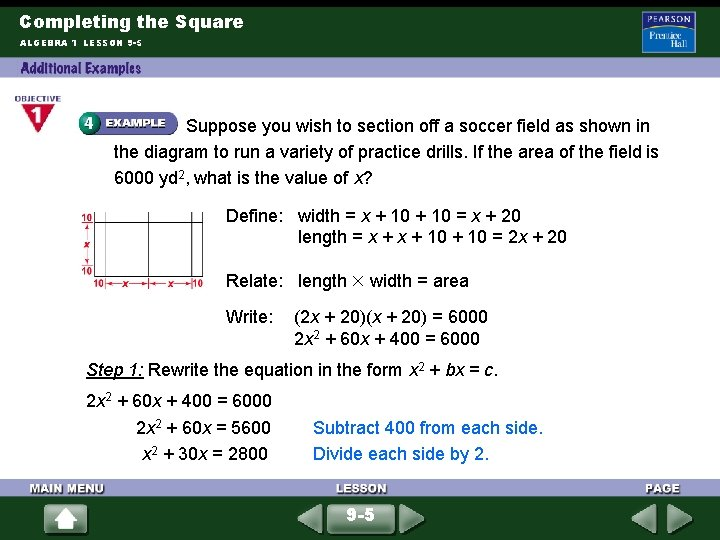Completing the Square ALGEBRA 1 LESSON 9 -5 Suppose you wish to section off a soccer field as shown in the diagram to run a variety of practice drills. If the area of the field is 6000 yd 2, what is the value of x? Define: width = x + 10 = x + 20 length = x + 10 = 2 x + 20 Relate: length width = area Write: (2 x + 20)(x + 20) = 6000 2 x 2 + 60 x + 400 = 6000 Step 1: Rewrite the equation in the form x 2 + bx = c. 2 x 2 + 60 x + 400 = 6000 2 x 2 + 60 x = 5600 x 2 + 30 x = 2800 Subtract 400 from each side. Divide each side by 2. 9 -5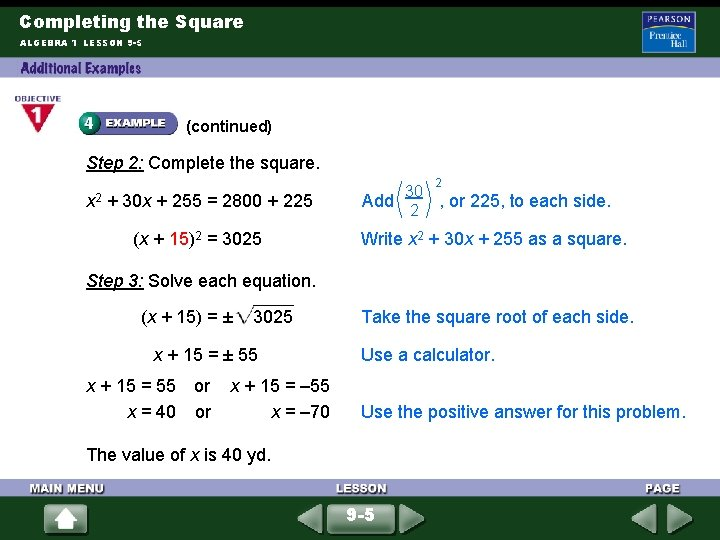Completing the Square ALGEBRA 1 LESSON 9 -5 (continued) Step 2: Complete the square. 2 x 2 + 30 x + 255 = 2800 + 225 (x + 15)2 = 3025 30 Add 2 , or 225, to each side. Write x 2 + 30 x + 255 as a square. Step 3: Solve each equation. (x + 15) = ± 3025 x + 15 = ± 55 x + 15 = 55 or x = 40 or x + 15 = – 55 x = – 70 Take the square root of each side. Use a calculator. Use the positive answer for this problem. The value of x is 40 yd. 9 -5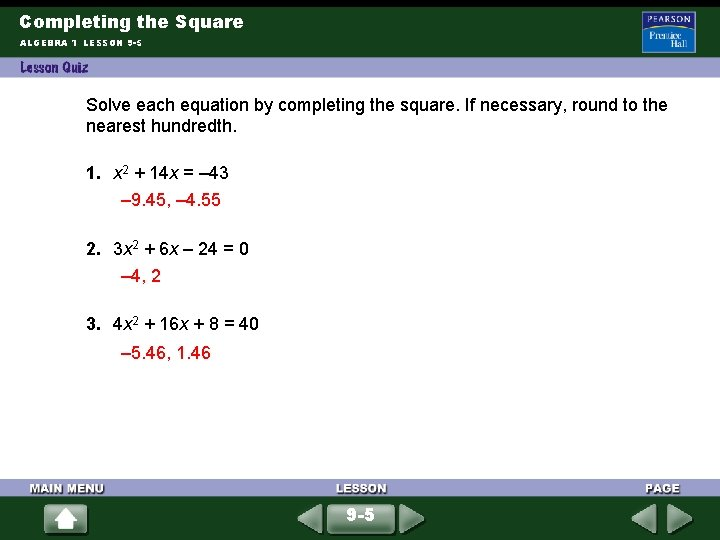Completing the Square ALGEBRA 1 LESSON 9 -5 Solve each equation by completing the square. If necessary, round to the nearest hundredth. 1. x 2 + 14 x = – 43 – 9. 45, – 4. 55 2. 3 x 2 + 6 x – 24 = 0 – 4, 2 3. 4 x 2 + 16 x + 8 = 40 – 5. 46, 1. 46 9 -5